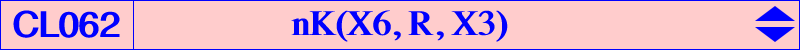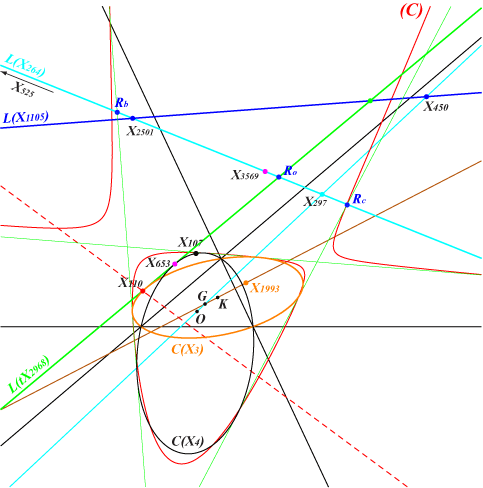CL062 is the class of isogonal nKs passing through the circumcenter O and the orthocenter H. They form a net of cubics that contains many special cubics. If the root R is u:v:w, the equation of the cubic is : ∑ u SA x (SB y - SC z)(c^2 SC y - b^2 SB z) = 0. The table gives a selection of these cubics with root R and centers other than O, H. CL062 is analogous to CL061, the class of cubics nK(X6, R, X2). The cubics in the table in the yellow lines are members of both classes, obtained when R lies on the line 107, 110, 648.cubic R centers X(3), X(4) and X(i) for i = remark K072 X(9969) 2, 6, 542, 842, 6328, 14246, 14355, 14356, 14357, 14366, 38940 focal K074 X(2) 4240, 14380 K105 X(1993) 15328, 15329 equilateral K164 X(2501) 468, 895, 3563, 3564, 6337, 14248 nK0, focal K165 X(10015) 1, 952, 953, 3109, 6790, 14260, 14887, 36944, 38941, 43692 strophoid K166 X(3569) 1316, 2698, 2782, 9513, 14251, 14382, 39641, 39642 focal K187 X(525) 30, 74, 34209, 34210, 39162, 39163, 39164, 39165, 42411, 42412, 46357, 46358 focal K383 X(14543) 1, 2, 6, 3945, 41501, 45926 cK K384 X(14544) 2, 6, 9, 40, 57, 84, 14550, 14551, 14552, 14553 K385 X(14546) 2, 6, 7, 55, 672, 673, 942, 943 K386 X(14545) 2, 6, 8, 56, 104, 517, 1193, 1220, 45998 K433 X(850) 23, 32, 67, 76, 98, 511, 43087 focal K932 X(3580) 110, 523, 7471, 14264, 15453, 15454 focal K1154 X(18314) 1141, 1154, 2070, 25043, 25044, 33565, 38896, 38897 focal K1180 X(24978) 5, 54, 14979, 32423, 38539, 38542 focal K1282 X(76)xX(230) 99, 512, 7468, 14265, 34157, 34175 focal X(1981) X(1), X(158), X(255), X(1982) cK X(4391) X(8), X(56), X(104), X(517), X(1325) focalClassification by type When the root lies on a certain locus (L), the cubic (K) = nK(X6, R, X3) has a specific characterization as shown in the next table and the figure below. L(M) denotes the trilinear polar of M and C(M) the circum-conic with perspector M.(L) characterization examples C(H) (K) decomposes into a line through H and its isogonal transform C(O) (K) decomposes into a line through O and its isogonal transform L(X1105) = 450,2501... (K) is a nK0 K164 L(X264) = 297,525... (K) is a focal cubic with focus on the circumcircle L(tX2968) = 651,653... (K) is a cK with node X(1), tX2968 = isotomic conjugate of X2968 extraversions of L(tX2968) (K) is a cK with node an excenterRemarks : • the line L(tX2968) and its extraversions (green lines) are the four common tangents to C(O) and C(H). • for any R on the (dashed red) line X110-X685, the cubic (K) also contains X(32), X(76), X(98), X(511). • when R is one of the intersections Ro, Ra, Rb, Rc of L(X264) with L(X1105) and extraversions, the cubic is a strophoid with node X(1) and excenters respectively. For example, nK(X6, Ro, X3) is K165. *** For any R on the (red) cubic (C), (K) has three concurring asymptotes. No ETC center was found on (C).Classification by centers Any cubic (K) = nK(X6, R, X3) that contains a center P (which is not O, H or an in/excenter) must contain the isogonal conjugate P* of P, P1 = OP /\ HP*, P2 = HP /\ OP* = P1*. See CL037 where P1 = Phi(P) and P2 = Psi(P). Note that each transformation Phi or Psi globally fixes any cubic (K). It follows that the cubics passing through P have 9 common points hence they belong to a same pencil whose root R lies on a certain line (L). (L) contains the trilinear poles of the lines OP, OP* (on the conic C(O)) and HP, HP* (on the conic C(H)) giving in general four decomposed cubics of the pencil. When R traverses (L), the tangents at P, P* to (K) intersect on the circum-conic passing through P and P*. Note that the four points P, P*, P1, P2 are not necessarily all distinct : • P = P1 (therefore P* = P2) if and only if P lies on the Orthocubic K006, in which case the tangents at P, P* concur at O. • P = P2 (therefore P* = P1) if and only if P lies on the McCay cubic K003, in which case the tangents at P, P* concur at H. In both cases, (K) has fixed tangents at P and P* for any root R on (L). This occurs in the yellow lines of the table.P P*, P1, P2 centers on (L) examples X(2) X(6) X107, X110, X648 X(7) X(55), X(942), X(943) X651 K385 X(8) X(56), X(104), X(517) X651 K386 X(9) X(57) X651, X1897 K384 X(10) X(58), X(573), X(13478) X110, X1897 X(13) X(15), X(17), X(61) X110 X(14) X(16), X(18), X(62) X110 X(32) X(76), X(98), X(511) X110, X685, X850 K433 X(39) X(83), X(182), X(262) X110Note : the cubics corresponding to the first line of the table are also members of the class CL061. *** Now, if P and Q are two triangle centers such that Q is not one of the 9 points mentioned above, let (L) and (L') be the two corresponding lines defined as above and intersecting at S. The cubic nK(X6, S, X3) already contains ten known triangle centers namely O, H, P, Q, P*, Q*, P1, Q1, P2 = P1*, Q2 = Q1*. These are all distinct when P and Q are not on the cubics K003 and K006. There are four more centers on the cubic namely S1 = PQ /\ P*Q*, S2 = S1* = PQ* /\ P*Q and their images under the transform Phi or Psi. The figure shows the cubic (K) passing through X(3), X(4), X(8), X(32), X(56), X(76), X(98), X(104), X(511), X(517), X(2703), X(2787) obtained with P = X(8) and Q = X(32) containing 12 identified centers.